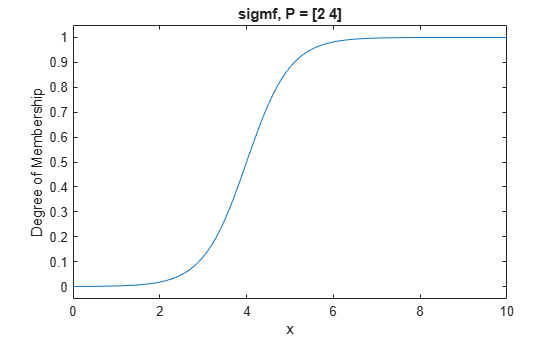# sigmf

Sigmoidal membership function

## Syntax

``y = sigmf(x,params)``

## Description

This function computes fuzzy membership values using a sigmoidal membership function. You can also compute this membership function using a `fismf` object. For more information, see fismf Object.

This membership function is related to the `dsigmf` and `psigmf` membership functions.

example

````y = sigmf(x,params)` returns fuzzy membership values computed using the sigmoidal membership function given by:$\text{\hspace{0.17em}}\text{\hspace{0.17em}}\text{\hspace{0.17em}}\text{\hspace{0.17em}}\text{\hspace{0.17em}}f\left(x;{a}_{k},{c}_{k}\right)=\frac{1}{1+{e}^{-{a}_{k}\left(x-{c}_{k}\right)}}$To define the membership function parameters, specify `params` as the vector [a c].Membership values are computed for each input value in `x`.```

## Examples

collapse all

Specify input values across the universe of discourse.

`x = 0:0.1:10;`

Evaluate membership function for the input values.

`y = sigmf(x,[2 4]);`

Plot the membership function.

```plot(x,y) title('sigmf, P = [2 4]') xlabel('x') ylabel('Degree of Membership') ylim([-0.05 1.05])```## Input Arguments

collapse all

Input values for which to compute membership values, specified as a scalar or vector.

Membership function parameters, specified as the vector [a c]. To open the membership function to the left or right, specify a negative or positive value for a, respectively. The magnitude of a controls the width of the transition area, and c defines the center of the transition area.

## Output Arguments

collapse all

Membership value returned as a scalar or a vector. The dimensions of `y` match the dimensions of `x`. Each element of `y` is the membership value computed for the corresponding element of `x`.

## Alternative Functionality

### `fismf` Object

You can create and evaluate a `fismf` object that implements the `sigmf` membership function.

```mf = fismf("sigmf",P); Y = evalmf(mf,X);```

Here, `X`, `P`, and `Y` correspond to the `x`, `params`, and `y` arguments of `sigmf`, respectively.

## Version History

Introduced before R2006a